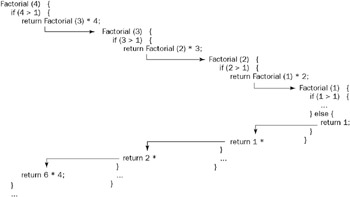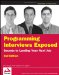# Chapter 7: Recursion

Recursion is a deceptively simple concept: Any routine that calls itself is recursive. Despite this apparent simplicity, understanding and applying recursion can be surprisingly complex. One of the major barriers to understanding recursion is that general descriptions tend to become highly theoretical, abstract, and mathematical. Although there is certainly value in that approach, this chapter will instead follow a more pragmatic course, focusing on example, application, and comparison of recursive and iterative (nonrecursive) algorithms.

## Understanding Recursion

Recursion is most useful for tasks that can be defined in terms of similar subtasks. For example, sort, search, and traversal problems often have simple recursive solutions. A recursive routine performs a task in part by calling itself to perform the subtasks. At some point, the routine encounters a subtask that it can perform without calling itself. This case, in which the routine does not recurse, is called the base case; the former, in which the routine calls itself to perform a subtask, is referred to as the recursive case.

 Tip Recursive algorithms have two types of cases: recursive cases and base cases.

These concepts can be illustrated with a simple and commonly used example: the factorial operator. n! (pronounced “n factorial”) is essentially the product of all integers between n and 1. For example, 4! = 4 _ 3 _ 2 _ 1 = 24. n! can be more formally defined as follows:

` n! = n (n – 1)! 0! = 1! = 1`

This definition leads easily to a recursive implementation of factorial. The task is determining the value of n!, and the subtask is determining the value of (n – 1)! In the recursive case, when n is greater than 1, the routine calls itself to determine the value of (n – 1)! and multiplies that by n. In the base case, when n is 0 or 1, the routine simply returns 1. Rendered in any C-like language, this looks like the following:

` int factorial( int n ){     if (n > 1) {  /* Recursive case */         return factorial(n-1) * n;     } else {        /* Base case */         return 1;     } }`

Figure 7-1 illustrates the operation of this routine when computing 4!. Notice that n decreases by 1 each time the routine recurses. This ensures that the base case will eventually be reached. If a routine is written incorrectly such that it does not always reach a base case, it will recurse infinitely. In practice, there is usually no such thing as infinite recursion: Eventually a stack overflow occurs and the program crashes - a similarly catastrophic event. (There is a form of recursion, called tail recursion, that can be optimized by the compiler to use the same stack frame for each recursive call. An appropriately optimized tail recursive algorithm could recurse infinitely because it wouldn’t overflow the stack.)Figure 7-1

 Tip Every recursive case must eventually lead to a base case.

This implementation of factorial represents an extremely simple example of a recursive routine. In many cases, your recursive routines may need additional data structures or an argument that tracks the recursion level. Often the best solution in such cases is to move the data structure or argument initialization code into a separate routine. This wrapper routine, which performs initialization and then calls the purely recursive routine, provides a clean, simple interface to the rest of the program.

For example, if you need a factorial routine that returns all of its intermediate results (factorials less than n), as well as the final result (n!), you most naturally return these results as an integer array, which means the routine needs to allocate an array. You also need to know where in the array each result should be written. These tasks are most easily accomplished using a wrapper routine, as follows:

` int[] allFactorials( int n ){ /* Wrapper routine */     int[] results = new int[ n == 0 ? 1 : n ];     doAllFactorials( n, results, 0 );     return results; } int doAllFactorials( int n, int[] results, int level ){     if( n > 1 ){  /* Recursive case */         results[level] = n * doAllFactorials( n - 1, results, level + 1 );         return results[level];     } else {        /* Base case */         results[level] = 1;         return 1;     } }`

You can see that using a wrapper routine enables you to hide the array allocation and recursion level tracking to keep the recursive routine very clean. In this case, it’s possible to determine the appropriate array index from n, avoiding the need for the level argument, but in many cases there is no alternative to tracking the recursion level as shown here.

 Tip It may be useful to write a separate wrapper routine to do initialization for a complex recursive routine.

Although recursion is a very powerful technique, it is not always the best approach, and rarely is it the most efficient approach. This is due to the relatively large overhead for routine calls on most platforms. For a simple recursive routine like factorial, many computer architectures spend more time on call overhead than the actual calculation. Iterative routines, which use looping constructs instead of recursive routine calls, do not suffer from this overhead and are frequently more efficient.

 Tip Iterative solutions are usually more efficient than recursive solutions.

Any problem that can be solved recursively can also be solved iteratively. Iterative algorithms are often quite easy to write, even for tasks that might appear to be fundamentally recursive. For example, an iterative implementation of factorial is relatively simple. It may be helpful to expand the definition of factorial, such that you describe n! as the product of every integer between n and 1, inclusive. You can use a for loop to iterate through these values and calculate the product:

` int factorial( int i ){     int n, val = 1;     for( n = i; n > 1; n-- )  /* n = 0 or 1 falls through */         val *= n;     return val; }`

This implementation is significantly more efficient than our previous recursive implementation because no routine calls are involved. Although it represents a different way of thinking about the problem, it’s not really any more difficult to write than the recursive implementation.

For some problems, there are no obvious iterative alternatives like the one just shown. It’s always possible, though, to implement a recursive algorithm without using recursive calls. Recursive calls are generally used to preserve the current values of local variables and restore them when the subtask performed by the recursive call is completed. Because local variables are allocated on the program’s stack, each recursive instance of the routine has a separate set of the local variables, so recursive calls implicitly store variable values on the program’s stack. You can therefore eliminate the need for recursive calls by allocating your own stack and manually storing and retrieving local variable values from this stack.

Implementing this type of stack-based interactive routine tends to be significantly more complicated than implementing an equivalent routine using recursive calls. In languages like Java or C#, a stack-based iterative implementation of a recursive algorithm won’t be more efficient than the recursive version. Given the large increase in complexity, you should implement recursive algorithms with recursive calls unless instructed otherwise. An example of a recursive algorithm implemented without recursive calls is given in the solution to the “Preorder Traversal, No Recursion” problem in Chapter 5.

 Tip A recursive algorithm can be implemented without recursive calls by using a stack, but it’s usually more trouble than it’s worth.

In an interview, a working solution is of primary importance; an efficient solution is secondary. Unless you’ve been told otherwise, go with whatever type of working solution comes to you first. If it’s a recursive solution, you might want to mention the inefficiencies inherent in recursive solutions to your interviewer, so it’s clear that you know about them. In the rare instance that you see a recursive solution and an iterative solution of roughly equal complexity, you should probably mention them both to the interviewer, indicating that you’re going to work out the iterative solution because it’s likely to be more efficient.Programming Interviews Exposed: Secrets to Landing Your Next Job, 2nd Edition (Programmer to Programmer)
ISBN: 047012167X
EAN: 2147483647
Year: 2007
Pages: 94

Similar book on Amazon

flylib.com © 2008-2017.
If you may any questions please contact us: flylib@qtcs.net